## Aspire's Library

A Place for Latest Exam wise Questions, Videos, Previous Year Papers,
Study Stuff for MCA Examinations

## NIMCET Previous Year Questions (PYQs)

#### NIMCET Probability PYQ

NIMCET PYQ
Given to events A and B such that odd in favour A are 2 : 1 and odd in favour of $A \cup B$ are 3 : 1. Consistent with this information the smallest and largest value for the probability of event B are given by

NIMCET Previous Year PYQNIMCET NIMCET 2023 PYQ

#### Solution

NIMCET PYQ
A bag contain different kind of balls in which 5 yellow, 4 black & 3 green balls. If 3 balls are drawn at random then find the probability that no black ball is chosen

NIMCET Previous Year PYQNIMCET NIMCET 2023 PYQ

#### Solution

NIMCET PYQ
Bag I contains 3 red, 4 black and 3 white balls and Bag II contains 2 red, 5 black and 2 white balls. One ballsis transferred from Bag I to Bag II and then a ball is drawn from Bag II. The ball so drawn is found to be black in colour. Then the probability, that the transferred is red, is:

NIMCET Previous Year PYQNIMCET NIMCET 2023 PYQ

#### Solution

NIMCET PYQ
A computer producing factory has only two plants T1 and T2 produces 20% and plant T2 produces 80% of the total computers produced. 7% of the computers produced in the factory turn out to be defective. It is known that P (computer turns out to be defective given that it is produced in plant T1 10P(computer turns out to be defective given that it is produced in plant T2 ). A computer produced in the factory is randomly selected and it does not turn out to be defective. Then the probability that it is produced in plant T2 is

NIMCET Previous Year PYQNIMCET NIMCET 2019 PYQ

#### Solution

NIMCET PYQ
Let U and V be two events of a sample space S and P(A) denote the probability of an event A. Which of the following statements is true?

NIMCET Previous Year PYQNIMCET NIMCET 2019 PYQ

#### Solution

NIMCET PYQ
If a man purchases a raffle ticket, he can win a first prize of Rs.5,000 or a second prize of Rs.2,000 with probabilities 0.001 and 0.003 respectively. What should be a fair price to pay for the ticket?

NIMCET Previous Year PYQNIMCET NIMCET 2019 PYQ

#### Solution

NIMCET PYQ
A man takes a step forward with probability 0.4 and backward with probability 0.6. The probability that at the end of eleven steps, he is one step away from the starting point is

NIMCET Previous Year PYQNIMCET NIMCET 2019 PYQ

#### Solution

NIMCET PYQ
Two numbers $a$ and $b$ are chosen are random from a set of the first 30 natural numbers, then the probability that $a^2 - b^2$ is divisible by 3 is

NIMCET Previous Year PYQNIMCET NIMCET 2019 PYQ

#### Solution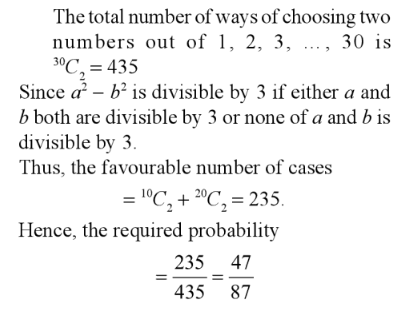NIMCET PYQ
If $0{\lt}P(A){\lt}1$ and $0{\lt}P(B){\lt}1$ and $P(A\cap B)=P(A)P(B)$, then

NIMCET Previous Year PYQNIMCET NIMCET 2022 PYQ

#### Solution

NIMCET PYQ
A four-digit number is formed using the digits 1, 2, 3, 4, 5 without repetition. The probability that is divisible by 3 is

NIMCET Previous Year PYQNIMCET NIMCET 2022 PYQ

#### Solution

NIMCET PYQ
There are two circles in xy −plane whose equations are $x^2+y^2-2y=0$ and $x^2+y^2-2y-3=0$. A point $(x,y)$ is chosen at random inside the larger circle. Then the probability that the point has been taken from smaller circle is

NIMCET Previous Year PYQNIMCET NIMCET 2022 PYQ

#### Solution

NIMCET PYQ
Two person A and B agree to meet 20 april 2018 between 6pm to 7pm with understanding that they will wait no longer than 20 minutes for the other. What is the probability that they meet?

NIMCET Previous Year PYQNIMCET NIMCET 2018 PYQ

#### Solution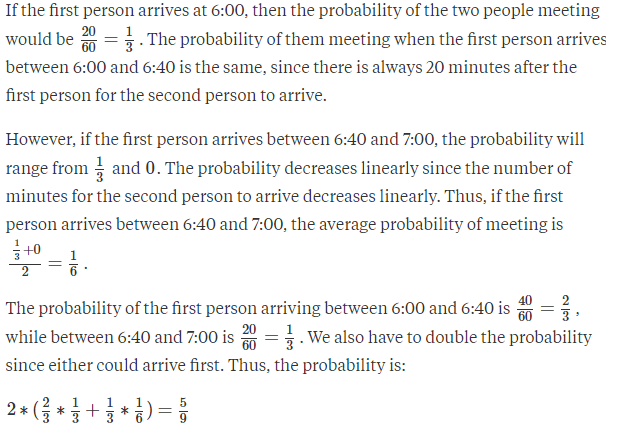NIMCET PYQ
Three numbers a,b and c are chosen at random (without replacement) from among the numbers 1, 2, 3, ..., 99. The probability that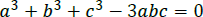is divisible by 3 is,

NIMCET Previous Year PYQNIMCET NIMCET 2018 PYQ

#### Solution

NIMCET PYQ
A and B play a game where each is asked to select a number from 1 to 25. If the two number match, both of them win a prize. The probability that they will not win a prize in a single trial is :

NIMCET Previous Year PYQNIMCET NIMCET 2018 PYQ

#### Solution

The total number of ways in which numbers can be choosed =25 x 25=625  The number of ways in which either players can choose same numbers = 25
Probability that they win a prize = 25/625 = 1/25
Thus, the probability that they will not win a prize in a single trial = 1 - 1/25 = 24/25

NIMCET PYQ
A and B are independent witness in a case. The chance that A speaks truth is x and B speaks truth is y. If A and B agree on certain statement, the probability that the statement is true is

NIMCET Previous Year PYQNIMCET NIMCET 2017 PYQ

#### Solution

P(A speaks truth) = x
P(B speaks truth) = y
Since, both A and B agree on certain  statement.
Hence, Total Probability =P(A)P(B)+P(A')P(B')
=xy  + (1-x)(1-y)
If statement is true then it means both A and B speaks truth.
∴ Required Probability =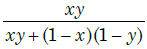NIMCET PYQ
In an entrance test there are multiple choice questions, with four possible answer to each question of which one is correct. The probability that a student knows the answer to a question is 90%. If the student gets the correct answer to a question, then the probability that he as guessing is

NIMCET Previous Year PYQNIMCET NIMCET 2017 PYQ

#### Solution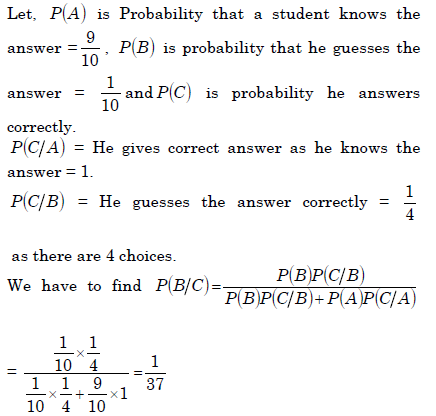NIMCET PYQ
A man is known to speak the truth 2 out of 3 times. He threw a dice cube with 1 to 6 on its faces and reports that it is 1. Then the probability that it is actually 1 is

NIMCET Previous Year PYQNIMCET NIMCET 2017 PYQ

#### Solution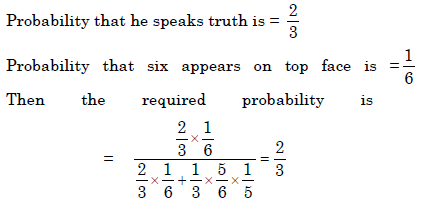NIMCET PYQ
Let A and B be two events such that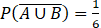,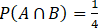and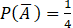where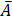stands for the complement of event A. Then the events A and B are

NIMCET Previous Year PYQNIMCET NIMCET 2017 PYQ

#### Solution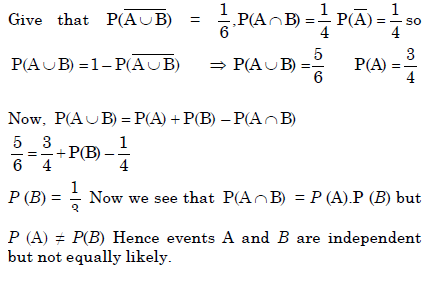NIMCET PYQ
If E1 and E2 are two events associated with a random experiment such that P (E2) = 0.35, P (E1 or E2) = 0.85 and P (E1 & E2) = 0.15 then P(E1) is

NIMCET Previous Year PYQNIMCET NIMCET 2017 PYQ

#### Solution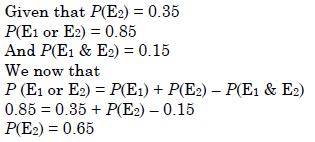NIMCET PYQ
The probability of occurrence of two events E and F are 0.25 and 0.50, respectively. the probability of their simultaneous occurrence is 0.14. the probability that neither E nor F occur is

NIMCET Previous Year PYQNIMCET NIMCET 2021 PYQ

#### Solution

NIMCET PYQ
If A and B are two events and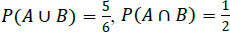, the A and B are two events which are

NIMCET Previous Year PYQNIMCET NIMCET 2018 PYQ

#### Solution

NIMCET PYQ
The probability that a man who is x years old will die in a year is p. Then, amongst n persons $A_1,A_2,\ldots A_n$ each x year old now, the probability that ${{A}}_1$ will die in one year and (be the first to die ) is

NIMCET Previous Year PYQNIMCET NIMCET 2021 PYQ

#### Solution

NIMCET PYQ
If a number x is selected at random from natural numbers 1,2,…,100, then the probability for $x+\frac{100}{x}{\gt}29$ is

NIMCET Previous Year PYQNIMCET NIMCET 2021 PYQ

#### Solution

NIMCET PYQ
If three thrown of three dice, the probability of throwing triplets not more than twice is

NIMCET Previous Year PYQNIMCET NIMCET 2021 PYQ

#### Solution

NIMCET PYQ
A problem in Mathematics is given to 3 students A, B, and C. If the probability of A solving the problem is 1/2 and B not solving it is 1/4 . The whole probability of the problem being solved is 63/64 , then what is the probability of solving it by C?

NIMCET Previous Year PYQNIMCET NIMCET 2020 PYQ

#### Solution

NIMCET PYQ
A and B play a game where each is asked to select a number from 1 to 25. If the two numbers match, both win a prize. The probability that they will not win a prize in a single trial is

NIMCET Previous Year PYQNIMCET NIMCET 2020 PYQ

#### Solution

Probability of winning a prize = $\frac{1}{25}$​
Thus, probability of not winning = $1-\frac{1}{25}=\frac{24}{25}$​

NIMCET PYQ
A computer producing factory has only two plants $T_1$ and $T_2$. Plant $T_1$ produces 20% and plant $T_2$ produces 80% of total computers produced. 7% of computers produced in the factory turn out to be defective. It is known that P (computer turns out to be defective given that it is produced in plant $T_1$) = 10P (computer turns out to be defective given that it is produced in plant $T_2$). where P(E) denotes the probability of an event E. A computer produced in the factory is randomly selected and it does not turn out to be defective. Then the probability that it is produced in plant $T_2$ is

NIMCET Previous Year PYQNIMCET NIMCET 2023 PYQ

#### Solution

NIMCET PYQ
A speaks truth in 60% and B speaks the truth in 50% cases. In what percentage of cases they are likely incontradict each other while narrating some incident is

NIMCET Previous Year PYQNIMCET NIMCET 2023 PYQ

#### NIMCET

Online Test Series,
and More.

#### NIMCET

Online Test Series,